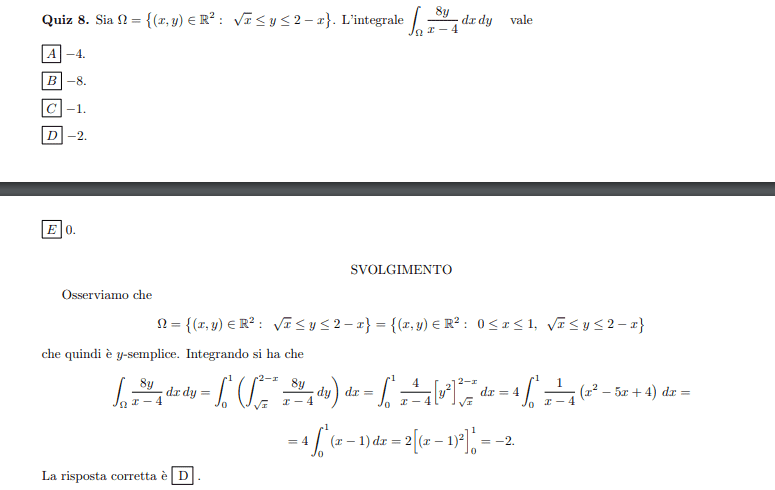# Question about a double integral region

Amaelle
Homework Statement:
look at the image
Relevant Equations:
double integral
Greetings All!

I have a problem finding the correct solution at first glance

My error was to determine the region of integration , for doing so I had to the intersection between y= sqrt(x) and y=2-x

to do so
x=(2-x)^2
to find at the end that x=1 or x=5

while graphically we can see that the region start from x=0 they intersect in x=1 and never meet again!

could someone help me with my confusion ?

Thank you!#### Attachments

Gold Member

•Amaelle
Amaelle
yes this i what I done
I just want to know why my analitical results was wrong

Mentor
2022 Award
Where exactly was your confusion? The region looks like this:
https://www.wolframalpha.com/input?i=root(x)+<+y+<+2-x
##x## is in ##[0,1]## and ##y## in ##[0,\sqrt{2}]##.

I don't see where you got ##x=5## from. Say ##t:=\sqrt{x}##. Then ##t^2+t-2=\left(t+1/2\right)^2-(1.5)^2\leq 0## and so ##0\leq t = \sqrt{x} \leq 1.5-0.5=1##.

Homework Helper
Gold Member
The solutions are x=1 and x=4 but the x=4 solution is not accepted because we want 2-x to be greater than zero. Remember that the inequality is $$0\leq \sqrt x\leq y\leq 2-x$$

•Amaelle
Amaelle
The solutions are x=1 and x=4 but the x=4 solution is not accepted because we want 2-x to be greater than zero. Remember that the inequality is $$0\leq \sqrt x\leq y\leq 2-x$$
thanks a million! you nail it!

•Delta2